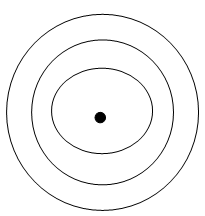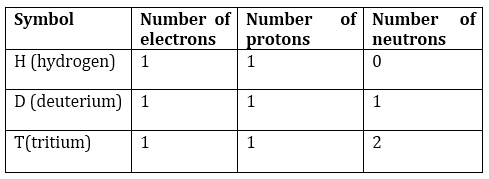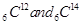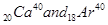1. /
2. CBSE
3. /
4. Class 09
5. /
6. Science
7. /
8. NCERT Solutions for Class...

# NCERT Solutions for Class 9 Science Structure of the Atom part 1

## NCERT Class 9 Science Chapter wise Solutions

• 01 –  Matter in Our Surroundings
• 02 – Is Mattter Around us Pure
• 03 – Atoms and Molecules
• 04 – Structure of the Atom
• 05 – The Fundamental Unit of Life
• 06 – Tissues
• 07 – Diversity in Living Organisms
• 08 – Motion
• 09 – Force and Laws of Motion
• 10 – Gravitation
• 11 – Word and Energy
• 12 – Sound
• 13 – Why Do We Fall Ill
• 14 – Natural Resources
• 15 – Improvement in Food Resources

## NCERT Solutions for Class 9 Science Structure of the Atom part 1

###### 1. What are canal rays?

Ans. E. Goldstein discovered positively charged rays, those rays are called canal rays.

###### 2. If an atom contains one electron and one proton, will it carry any charge or not?

Ans. That atom will not contain any charge because one negative charge of single electron and one positive charge of single proton are neutralized by each other.

NCERT Solutions for Class 9 Science Structure of the Atom part 1

###### 1. On the basis of Thomson’s model of an atom, explain how the atom is neutral as a whole.

Ans. As per Thomson’s model of an atom, the number of electrons (negatively charged) are equal to the number of protons (positively charged) in an atom. Hence the + and – charges are neutralized by each other that makes atom neutral as a whole.

###### 2. On the basis of Rutherford’s model of an atom, which subatomic particle is present in the nucleus of an atom?

Ans. The sub atomic particle proton is present in the nucleus of an atom according to Rutherford’s model of an atom.

NCERT Solutions for Class 9 Science Structure of the Atom part 1

###### 3. Draw a sketch of Bohr’s model of an atom with three shells.

Ans.###### 4. What do you think would be the observation if the α-particle scattering experiment is carried out using a foil of a metal other than gold?

Ans. Yes the observations would have been different if the α-particle scattering experiment is carried out using a foil of a metal other than gold.

###### 5. Name the three sub-atomic particles of an atom.

Ans. Proton, Neutron and Electron.

###### 6. Helium atom has an atomic mass of 4 u and two protons in its nucleus. How many neutrons does it have?

Ans. The number of neutrons present in a helium atom

= atomic mass – no. of protons

= 4-2 = 2

NCERT Solutions for Class 9 Science Structure of the Atom part 1

###### 1. Write the distribution of electrons in carbon and sodium atoms.

Ans. Distribution of electrons in carbon atom: atomic number of carbon = 6 = 2,4

Distribution of electrons in sodium atom: atomic number of sodium = 11 = 2,8,1

###### 2. If K and L shells of an atom are full, then what would be the total number of electrons in the atom?

Ans. If K and L shells of an atom are full, then the total number of electron in the atom will be 10 because K shell can accommodate total 2 and L shell can accommodate maximum 8 electrons that makes a total of 10.

NCERT Solutions for Class 9 Science Structure of the Atom part 1

###### 1. How will you find the valency of chlorine, sulphur and magnesium?

Ans. The electrons present in the outermost shell of an atom are known as the valence electrons. Those electrons determine the valency of that atom.

The atomic number of chlorine is 17 = 2,8,7

so the number of valence electrons for chlorine is 7 and it needs 1 more electron to complete its octet (8). Therefore, its valency is one.

similarly, Sulphur = 16 = 2,8,6

so the number of valence electrons for sulphur is 6 and it needs 2 more electrons to complete its octet (8). Therefore, its valency is two.

similarly, for magnesium = 12 = 2,8,2

It becomes easier for magnesium to give away its two valence than to acquire 6 more therefore its valency is two.

###### 2. If number of electrons in an atom is 8 and number of protons is also 8, then

(i) what is the atomic number of the atom? and

(ii) what is the charge on the atom?

Ans. (i) atomic number = number of protons = number of electrons = 8

(ii)atom will be neutral (no charge) because number of protons(+) is equal to the number of electrons (-).

###### 3. With the help of Table 4.1, find out the mass number of oxygen and sulphur atom.

Ans. mass number of oxygen = number of neutrons + number of protons = 8+8 =16

mass number of sulphur = number of neutrons + number of protons = 16 + 16 = 32

NCERT Solutions for Class 9 Science Structure of the Atom part 1

###### 1. For the symbol H,D and T tabulate three sub-atomic particles found in each of them.

Ans.###### 2. Write the electronic configuration of any one pair of isotopes and isobars.

Ans. Isotopes of carbon:both have same number of electrons and protons.

Isobars:## NCERT Solutions for Class 9 Science

NCERT Solutions Class 9 Science PDF (Download) Free from myCBSEguide app and myCBSEguide website. Ncert solution class 9 Science includes text book solutions .NCERT Solutions for CBSE Class 9 Science have total 15 chapters. 9 Science NCERT Solutions in PDF for free Download on our website. Ncert Science class 9 solutions PDF and Science ncert class 9 PDF solutions with latest modifications and as per the latest CBSE syllabus are only available in myCBSEguide.

## CBSE app for Class 9

To download NCERT Solutions for class 9 Science, Computer Science, Home Science,Hindi ,English, Maths Social Science do check myCBSEguide app or website. myCBSEguide provides sample papers with solution, test papers for chapter-wise practice, NCERT solutions, NCERT Exemplar solutions, quick revision notes for ready reference, CBSE guess papers and CBSE important question papers. Sample Paper all are made available through the best app for CBSE students and myCBSEguide website.### Test Generator

Create question paper PDF and online tests with your own name & logo in minutes.### myCBSEguide

Question Bank, Mock Tests, Exam Papers, NCERT Solutions, Sample Papers, Notes